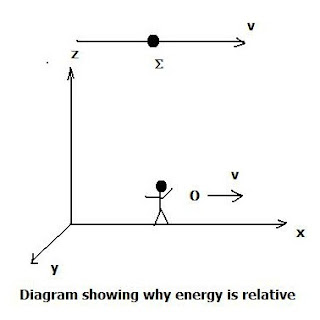## Friday, June 8, 2018

### Selected Questions- Answers From All Experts Astronomy Forum (Proper Time)

Question:  While reading a book on special relativity I came across the term proper time. Can you explain what it is, how used?

Proper time is a time defined in terms of the relativistic interval (from special relativity):

g   =        [1 – (v/c) 2] -1/2

where v is a specific velocity- say of an observer’s frame, c is the speed of light.

Then the proper time is: dt(p) = dt/ g

Why use proper time? Basically, because normal time measures are unreliable and subjective owing to relativity. For example, even saying or asserting the energy is greater or less at one time (t2) than time (t1) is impossible since energy (like mass, time, length) varies depending on the observer’s frame.

For example, consider the energy of an object (denoted by the Greek letter sigma) moving at velocity v in the simple diagram below:But, to the observer O moving in a reference frame with the same velocity, the object appears at rest so its kinetic energy   KE= 0. In more technical consideration, on the basis of the Lorentz transformation (google!) the energy E’ in a new frame will depend on the energy E in an older frame according to:

E’ =    g o  (E –   v o   p x )

Where   v  is the velocity and p x  the momentum in direction (x).

The factor  g o   =      m o v/  (1 - v2/ c2 ) -1/2

and   m  is the rest mass.

The point is that the energy in the new frame E depends on the momentum in the old frame, p x. If this momentum is not conserved, then energy won’t be conserved in the new frame.

Bottom line, unless one employs corrections using proper time to make energy estimates or statements, he will end up talking or writing nonsense.

For example, let’s say you are looking at an object receding at a velocity of 0.5 c, and assert based on careful ordinary time measurements that it emerged 1 billion years after the Big Bang. Or  12.7 billion year removed from your observation. How does this compare to the proper time?

We have: dt(p) = dt/ g

Using a finite difference equation:

D t(p) =  D t / g

D t= 12.7 billion yrs.  where:

= [1 – (0.5c/c)2 ]  -1/2   = [1 – (0.25)]  -1/2   = [0.75]  -1/2  = 1.155

Then the proper time interval (finite difference result)  is  D t (p) =

(12.7 x 10 )/1.155 = 1.1 x 10 10       yrs.

Or 11 billion years not 12.7 billion.

This obviously also means that one cannot say or assert that from the Big Bang there has been “an infinite” amount of time, or instants. No, there hasn’t. One needs to do the computation for the actual time interval using the proper time format.